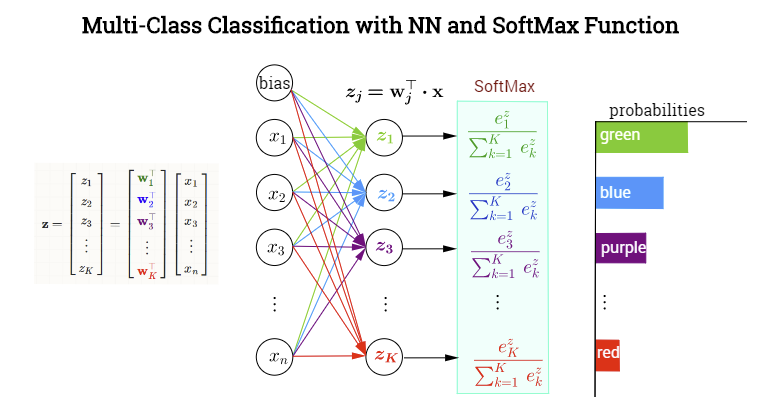#### SOFTMAX ACTIVATION:

This is a good resource.

In multiclass classification networks the softmax function:

The last hidden layer produces output values forming a vector $$\vec x = \mathbf x$$. The output neuronal layer is meant to classify among $$K=1,\dots,k$$ categories with a SoftMax activation function assigning conditional probabilities (given $$\mathbf x$$) to each one the $$K$$ categories. In each node in the final (or ouput) layer the pre-activated values (logit values) will consist of the scalar products $$\mathbf{w}_j^\top\mathbf{x}$$, where $$\mathbf w_j\in\{\mathbf{w}_1, \mathbf{w}_2,\dots,\mathbf{w}_k\}$$. In other words, each category, $$k$$ will have a different vector of weights pointing at it, determining the contribution of each element in the output of the previous layer (including a bias), encapsulated in $$\mathbf x$$. However, the activation of this final layer will not take place element-wise (as for example with a sigmoid function in each neuron), but rather through the application of a SoftMax function, which will map a vector in $$\mathbb R^k$$ to a vector of $$K$$ elements in $$[0,1]$$. Here is a made-up NN to classify colors:The softmax as

$\sigma(j)=\frac{\exp(\mathbf{w}_j^\top \mathbf x)}{\sum_{k=1}^K \exp(\mathbf{w}_k^\top\mathbf x)}=\frac{\exp(z_j)}{\sum_{k=1}^K \exp(z_k)}$

This will result in a normalization of the output adding up to $$1$$, interpretable as a probability mass functionn.

From Wikipedia:

"In probability theory, the output of the softmax function can be used to represent a categorical distribution – that is, a probability distribution over K different possible outcomes."

Notice that there is truly no strict need for an activation function. As in this post:

"At the end of a network, you can either use nothing (logits) and get a multi parameter regression." 

So why do it?

1. softmax is optimal for maximum-likelihood estimation of the model parameters.

2. The properties of softmax (all output values in the range (0, 1) and sum up to 1.0) make it suitable for a probabilistic interpretation that’s very useful in machine learning.

3. Softmax normalization is a way of reducing the influence of extreme values or outliers in the data without removing data points from the set.

Although we can use mean squared error, cross-entropy is the preferred loss function for classification NN with softmax activation in the last layer. It is given by the function:

$\begin{eqnarray} C = -\frac{1}{k} \sum_{k=1}^K \left[y_k \log (\sigma(z_k)) + (1-y_k ) \log (1-\sigma(z_k)) \right] \end{eqnarray}$

As explained here, average cross-entropy (ACE) would be calculated as:

computed       | targets              | correct?
-----------------------------------------------
0.3  0.3  0.4  | 0  0  1 (democrat)   | yes
0.3  0.4  0.3  | 0  1  0 (republican) | yes
0.1  0.2  0.7  | 1  0  0 (other)      | no

$-\left( (\log(0.3)\times 0) + (\log (0.3)\times 0) + (\log (0.4)\times1) \right) = -\log(0.4)$

With one-hot encoding, the $$y$$ vector will eliminate all elements, except for one (the correct value for the example). The expression $$y_k\log \hat y_k + (1-y_k)\log(1-\hat y_k)$$ will become $$\log \hat y_k$$ so that if the calculated probability for that category is close to $$1$$, the loss function will approach zero, whereas, if the probabily (output of the softmax for that category) is close to zero, the loss will tend to infinity.

##### Derivative of the softmax function with respect to the logit $$(z_j =\mathbf W_j^\top \cdot \mathbf x)$$:

Computing the

$\frac{\partial}{\partial z_i}\sigma(j)=\frac{\partial}{\partial z_i}\frac{\exp(z_j)}{\sum_{k=1}^K \exp(z_k)}$

The derivative of $$\sum_{k=1}^K \exp(z_k)$$ with respect to any $$z_i$$ will be $$\exp(z_i)$$. As for the numerator, $$\exp(z_j)$$ the derivative will be $$\exp(z_i)$$ if and only if $$z_i = z_j$$; otherwise the derivative is $$0$$.

If $$i = j$$, and using the quotient rule,

\begin{align}\frac{\partial}{\partial z_i}\frac{\exp(z_j)}{\sum_{k=1}^K \exp(z_k)} &= \frac{\mathbf \exp(z_j)\sum_{k=1}^K \exp(z_k)\quad - \exp(z_i)\exp(z_j)}{\left[\sum_{k=1}^K \exp(z_k)\right]^2}\\[2ex] &= \frac{\exp(z_j)}{\sum_{k=1}^K \exp(z_k)}\frac{\sum_{k=1}^K \exp(z_k)-\exp(z_i)}{\sum_{k=1}^K \exp(z_k)}\\[2ex] &=\sigma(z_j)\,(1 - \sigma(z_i)) \end{align}

If on the other hand, $$i \neq j$$:

\begin{align}\frac{\partial}{\partial z_i}\frac{\exp(z_j)}{\sum_{k=1}^K \exp(z_k)} &= \frac{0\quad - \exp(z_i)\exp( z_j)}{\left[\sum_{k=1}^K \exp(z_k)\right]^2}\\[2ex] &= - \frac{\exp(z_j)}{\sum_{k=1}^K \exp(z_k)}\frac{\exp(z_i)}{\sum_{k=1}^K \exp(z_k)}\\[2ex] &=-\sigma(z_j)\,\sigma(z_i) \end{align}

These two scenarios can be brought together as

$\frac{\partial}{\partial z_i} \sigma(z_j)= \sigma(z_j)\left(\delta_{ij}-\sigma(z_i)\right)$

MIT Deep Learning book